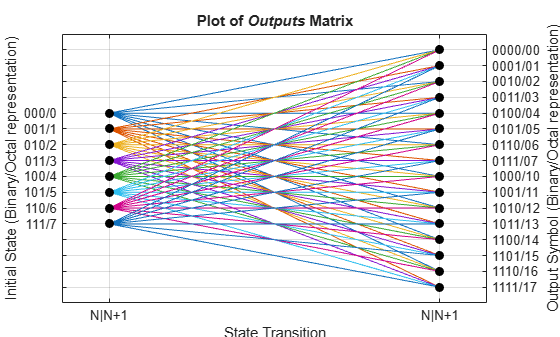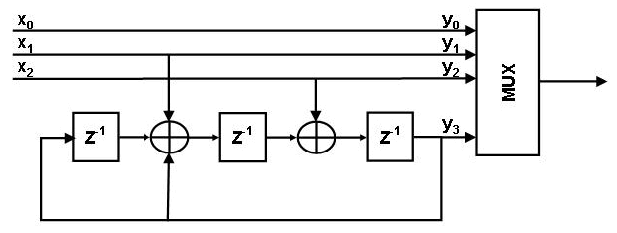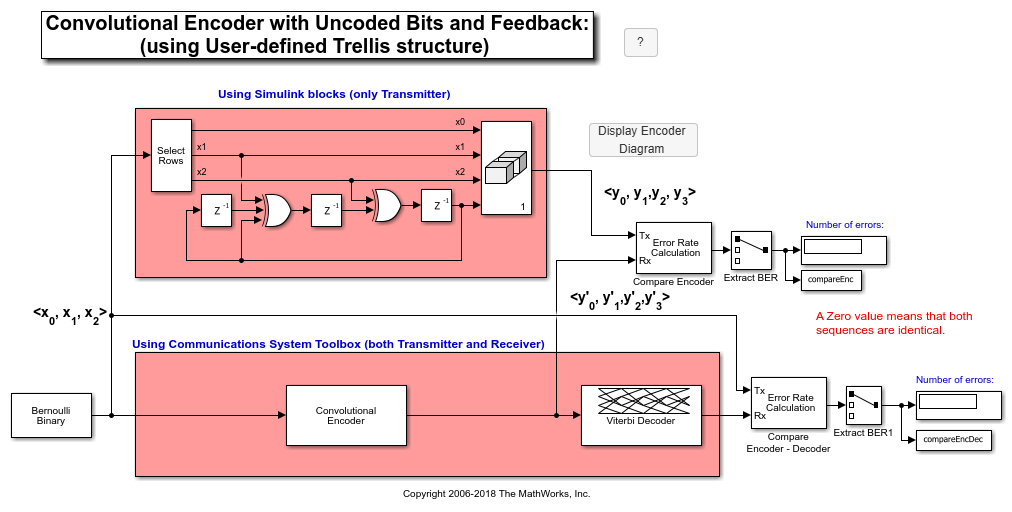## Creation, Validation, and Testing of User Defined Trellis Structure

### Create User Defined Trellis Structure

This example demonstrates creation of a nonstandard trellis structure for a convolutional encoder with uncoded bits and feedback. The encoder cannot be created using `poly2trellis` because the peculiar specifications for the encoder do not match the input requirements of `poly2trellis`.

You can manually create the trellis structure, and then use it as the input trellis structure for an encoder and decoder. The Convolutional Encoder and Viterbi Decoder blocks used in the Convolutional Encoder with Uncoded Bits and Feedback model load the trellis structure created here using a `PreLoadFcn` callback.

Convolutional Encoder

Create a rate 3/4 convolutional encoder with feedback connection whose MSB bit remains uncoded.Declare variables according to the specifications.

```K = 3; N = 4; constraintLength = 4; ```

Create trellis structure

A trellis is represented by a structure with the following fields:

• `numInputSymbols` – Number of input symbols

• `numOutputSymbols` – Number of output symbols

• `numStates` – Number of states

• `nextStates` – Next state matrix

• `outputs` – Output matrix

For more information about these structure fields, see `istrellis`.

Reset any previous occurrence of `myTrellis` structure.

`clear myTrellis;`

Define the trellis structure fields.

```myTrellis.numInputSymbols = 2^K; myTrellis.numOutputSymbols = 2^N; myTrellis.numStates = 2^(constraintLength-1);```

Create `nextStates` Matrix

The `nextStates` matrix is a [`numStates` x `numInputSymbols`] matrix. The (i,j) element of the next state matrix is the resulting final state index that corresponds to a transition from the initial state i for an input equal to j.

```myTrellis.nextStates = [0 1 2 3 0 1 2 3; ... 6 7 4 5 6 7 4 5; ... 1 0 3 2 1 0 3 2; ... 7 6 5 4 7 6 5 4; ... 2 3 0 1 2 3 0 1; ... 4 5 6 7 4 5 6 7; ... 3 2 1 0 3 2 1 0; ... 5 4 7 6 5 4 7 6] ```
```myTrellis = struct with fields: numInputSymbols: 8 numOutputSymbols: 16 numStates: 8 nextStates: [8x8 double] ```

Plot `nextStates` Matrix

Use the `commcnv_plotnextstates` helper function to plot the `nextStates` matrix to illustrate the branch transitions between different states for a given input.

`commcnv_plotnextstates(myTrellis.nextStates);`Create` outputs `Matrix

The `outputs` matrix is a [`numStates` x `numInputSymbols`] matrix. The (i,j) element of the output matrix is the output symbol in octal format given a current state i for an input equal to j.

```outputs = [0 2 4 6 10 12 14 16; ... 1 3 5 7 11 13 15 17; ... 0 2 4 6 10 12 14 16; ... 1 3 5 7 11 13 15 17; ... 0 2 4 6 10 12 14 16; ... 1 3 5 7 11 13 15 17; ... 0 2 4 6 10 12 14 16; ... 1 3 5 7 11 13 15 17]```
```outputs = 8×8 0 2 4 6 10 12 14 16 1 3 5 7 11 13 15 17 0 2 4 6 10 12 14 16 1 3 5 7 11 13 15 17 0 2 4 6 10 12 14 16 1 3 5 7 11 13 15 17 0 2 4 6 10 12 14 16 1 3 5 7 11 13 15 17 ```

Use `oct2dec` to display these values in decimal format.

`outputs_dec = oct2dec(outputs)`
```outputs_dec = 8×8 0 2 4 6 8 10 12 14 1 3 5 7 9 11 13 15 0 2 4 6 8 10 12 14 1 3 5 7 9 11 13 15 0 2 4 6 8 10 12 14 1 3 5 7 9 11 13 15 0 2 4 6 8 10 12 14 1 3 5 7 9 11 13 15 ```

Copy outputs matrix into the `myTrellis` structure.

`myTrellis.outputs = outputs`
```myTrellis = struct with fields: numInputSymbols: 8 numOutputSymbols: 16 numStates: 8 nextStates: [8x8 double] outputs: [8x8 double] ```

Plot `outputs` Matrix

Use the `commcnv_plotoutputs` helper function to plot the `outputs` matrix to illustrate the possible output symbols for a given state depending on the input symbol.

`commcnv_plotoutputs(myTrellis.outputs, myTrellis.numOutputSymbols);`Check Resulting Trellis Structure

`istrellis(myTrellis)`
```ans = logical 1 ```

A return value of `1` confirms the trellis structure is valid.

### Convolutional Encoder with Uncoded Bits and Feedback

The model serves as a unit test bench for the convolutional code implemented. The model shows how to define and use a trellis that describes a convolutional code. The particular code in this example cannot be described by a set of generator and feedback connection polynomials. The code's trellis cannot be created by the `poly2trellis` because that function expects generator and feedback connection polynomials as input arguments.

Structure of the Convolutional Code

This figure shows the convolutional code.Structure of the Example

The major components in this example include:

• A transmit path that builds a representation of the convolutional encoder using discrete low-level delay and sum (XOR) blocks. This representation looks very similar to the figure showing the structure of the convolutional code.

• A transmit-receive path that builds a representation of the same convolutional encoder using the Convolutional Encoder block. In this case, the description of the encoder is within the block's Trellis structure parameter. This portion of the model also includes the Viterbi Decoder block, which decodes the convolutional code.

• Both paths compute the number of bit errors.

Open Example Model and Explore Its Contents

Open the example model `slex_commcnvencoder`.Results and Displays

When you run the simulation, the block labeled `Compare Encoder` checks that the two representations of the encoder yield the same result. The block labeled `Compare Encoder - Decoder` checks that the encoder and decoder work properly as a pair. Each Display block in the model shows an error rate of zero, as expected.

```Error rate for Compare Encoder signal: 0.000 Error rate for Compare Encoder-Decoder signal: 0.000 ```